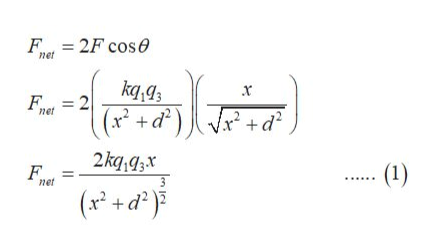Question
141 views

In the figure particles 1 and 2 of charge q1 = q2 = +22.40 × 10-19 C are on a y axis at distance d = 19.0 cm from the origin. Particle 3 of charge q3 = +20.80 × 10-19 C is moved gradually along the x axis from x = 0 to x = +6.88 m. At what values of x will the magnitude of the electrostatic force on the third particle from the other two particles be

(a) minimum and

(b) maximum?

What are the (c) minimum and (d) maximum magnitudes?

check_circle

Step 1

Let, F1 and F2 be the force at the location of charge 3, due to charge 1 and 2 respectively.

Let, x be the distance from the origin to the position of charge 3.

Since, the magnitude of charges on charge 1 and 2 are equal, F1 and F2 will also become equal.

F1 = F2=F

Step 2

Force due to charge 1 and charge 2 on charge 3 is,

Step 3

At a distance x from the origin, where charge ...help_outlineImage TranscriptioncloseF 2F cose net kq,a3 x+d) +d x net 2kq,gzx (1) net (x +d fullscreen

### Want to see the full answer?

See Solution

#### Want to see this answer and more?

Solutions are written by subject experts who are available 24/7. Questions are typically answered within 1 hour.*

See Solution
*Response times may vary by subject and question.
Tagged in

### Physics